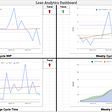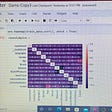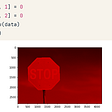# Genetic Algorithms for Optimization

Genetic algorithms have gained popularity recently owing to its similarity to genetic evolution and use in optimization techniques. Prof John Holland, known as the father of genetic algorithms, presented the concept in 1960 at the University of Michigan. The idea was mainly inspired by Charles Darwin`s theory of Evolution. These algorithms are widely used in computational mathematics nowadays.

Genetic algorithms are a kind of meta-heuristics which does not guarantee an optimal solution. Heuristics are algorithms that perform a search to find the best solution for problems that cannot be solved directly such as Non-Polynomial (NP) problems. It uses biological operators such as selection, crossover, and mutation. In this article, we are going to describe how a guided search helps these algorithms to evolve to the best solution. Genetic Algorithm is an evolutionary algorithm which can be categorized into population-based & memetic algorithms. Additionally, It can also be used for NP-Complete problems like Travelling salesman problem.

# Where did they come from?

Charles Darwin in his book “The origin of species” concluded his 20 years of research that how humans had evolved through time. It generalizes the idea of Evolution and presents the theory of Natural Selection. It emphasizes the survival of fittest through generations. The modern genomic tells us that every human holds 3 billion nucleotides. (long enough to be written on 262K A4 pages).

Let`s look at a small analogy of how “YOU” came up to be the best of others. A simple pyramid theory describes it is a way that you have two parents and those two parents have 4 ancestors. This pedigree collapse tells If you move this chain further to 40 generations keeping an average generation gap of 25 years then you have nearly 2 Billion forefathers, those who survived earthquakes, rains, drought, wars, and diseases. Therefore, I am happy that the product of two Billion fittest people that ever survived on the planet is reading this article.

# How to use this evolution for Programming?

Now, let’s use the same theory from upside down as the Inverse pyramid theory in which we have millions of possible solutions for any problem and we want to choose best among them as parents and create offspring by mutation to come up fittest solution after a few generations.

This algorithm can be conceived in a tree-like representation. A GA has the following phases

1. Initial Population
2. Fitness Function
3. Selection
4. Crossover
5. Mutation

Every human has a smaller mutation compared to others even in identical children. 99% of human DNA resembles every other human. Additionally, 96% of our DNA resembles chimpanzees, 90%, 80% with cats and cows respectively.

## 1. Genesis (Initializing Population)

For every heuristic, we anticipate some random solution to check the function value and then perform a gradient search or simulated annealing to check where we are leading. For a mathematical problem, we always have few variables and those variables have often some constraints or ranges. Higher the population is easier it is to converge to a solution but storing big sets of values can be memory constrained in some systems.

`# low = lower range for variables, high = higher range for variablesdef genesis(size,var, high , low):   pop_size = (size,var)   new_pop = np.random.uniform(low=low,high=high,size=pop_size)   return new_pop`

## 2. Fitness Function

It is a function that scores every solution to help in choosing the best solution. This fitness function is a mathematical equation that we want to maximize or minimize. A complex fitness function can create higher computational costs in case of a bigger population.

`def fitness(p):# Evaluating fitness Interference function "double fit (doublep[])".   fitness=np.zeros((len(p),1))   for i in range(len(p)):       x,y,z = p[i] , p[i] , p[i]       fitness[i,0] = 2*x*z*np.exp(-x) - 2*y**3 + y**2 -3*z**3   return fitness`

## 3. Selection

After using a scoring criterion to find the fittest we use a few parents to breed the next generation. More parents mean more diversity in solution and It becomes easier to reach an optimal solution.

`def selection(pop, fitness, num_parents):   parents = np.empty((num_parents, pop.shape))   for parent_num in range(num_parents):      max_fitness_idx = np.where(fitness == np.max(fitness))      max_fitness_idx = max_fitness_idx      parents[parent_num, :] = pop[max_fitness_idx, :]      fitness[max_fitness_idx] = -99999999999   return parents`

## 4. Crossover

This operation is performed to create a blend and avoid repeating the same solution. It is like natural selection where people living in different areas adopt different skin and hair colors. Crossover has different types such as one point, two-point, and uniform.

`def crossover(parents, offspring_size):   offspring = np.empty(offspring_size)   crossover_point = np.uint8(offspring_size/2)   for k in range(offspring_size):      parent1_idx = k%parents.shape      parent2_idx = (k+1)%parents.shape      offspring[k, 0:crossover_point] = parents[parent1_idx,     0:crossover_point]      offspring[k, crossover_point:] = parents[parent2_idx, crossover_point:]   return offspring`

## 5. Mutation

The word Mutants is now quite famous from the movie Xmen where every person has a distinct quality. We initialize mutation to increase diversity in solution. The mutation rate governs how much change we want to introduce in the population. In human DNA, the identical twin may be mutated from 0.1% to 0.4%. There are different types of mutation such as bit flip, swap, inverse, uniform, nonuniform, Gaussian, shrink, and others.

`def mutation(offspring_crossover):   for idx in range(offspring_crossover.shape):      random_value = np.random.uniform(0, 10, 1)# 10 percent change maximum keeping 90 percent mutation rate       i= randint(0, 2)      offspring_crossover[idx, i] = offspring_crossover[idx, i] + random_value    return offspring_crossover`

# An Illustration of Optimization:

We want to maximize this equation.

Note: We can minimize a function by simple making it negative with the same code i.e: g(x) = -f(x)

We have three variables x,y,z and we are choosing these parameters for Solution:

a) 50 Generation

b) Mutation Rate: 10%

c) Parent: 2

d) Mutated Off Spring: 4

e) Initial Population: 100k

`import numpy as npfrom random import randintnum_var = 3sizeof_pop= 100000upper_limit = 100lower_limit = 0num_parents = 2population = genesis(sizeof_pop , num_var, upper_limit ,lower_limit)print(population)Scores=[]num_generations = 50for generation in range(num_generations):   print("\n\nGeneration : ", generation)   fitness_score = fitness(population)   parents = selection(population, fitness_score, num_parents)   print("Best Parents \n",parents)   offspring_crossover = crossover(parents, offspring_size=(2*num_parents, num_var))   offspring_mutation = mutation(offspring_crossover)   print("Mutated OffSprings\n",offspring_mutation)   population[0:parents.shape, :] = parents   population[parents.shape:6, :] = offspring_mutation   Scores.append(np.max(fitness_score))   print("Best result : ", np.max(fitness_score))`

This will run the algorithm for 50 generations and display the results. A colab version is available here.

`fitness = fitness(population)best_match_idx = np.where(fitness == np.max(fitness))print("P : ", population[best_match_idx, :])print(" Value for  x= {}".format(population[best_match_idx, :]))print(" Value for  y= {}".format(population[best_match_idx, :]))print(" Value for  z= {}".format(population[best_match_idx, :]))print("Best solution fitness : ", fitness[best_match_idx])`

We can find different values of a variable in every run, as described earlier it is not a deterministic algorithm.

`import matplotlib.pyplot as pltEvaluations = Scoresplt.plot(Evaluations,'o-',color='red')plt.xlabel('Generations')plt.ylabel('Function Value')plt.title("Maximization of Function")plt.grid(True)plt.show()`

A similar task can be performed with Matlab using a global optimization toolbox.

The code can be found here.

--

--

--

## More from Analytics Vidhya

Analytics Vidhya is a community of Analytics and Data Science professionals. We are building the next-gen data science ecosystem https://www.analyticsvidhya.com

## Understanding Singular Value Decomposition and its Application in Data Science## Customer Journey Mapping — Where to begin — Part 3 What and How to Measure## Flow Metrics In Five Minutes## In Class Hackathon : My approach to exploratory data analysis## 3 Captivating Questions to Ask at the End of Your Data Science Interview## Herding Model in Financial Markets## Unleash the power of your data## Neel K.

Machine Learning and Networking Engineering

## Image processing with Keres in Python- Part 1## [edX SDS Micromasters] 6.86x Machine Learning with Python-From Linear Models to Deep Learning## Introduction Python Machine Learning## What is Reinforcement Learning?